# MPL115A fixed point question

cancel
Showing results for
Did you mean:

## MPL115A fixed point question

6,143 Views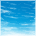Contributor III
I am trying to make sense of the coefficients I receive from the pressure sensor MPL115A.  I can successfully access the IIC bus and get the data, but the algorithm provided in the AN3785 does not appear to work (at least for me).  Closer inspection of the comments raises some questions.  For example, the coeff C11 is described as a signed number with no int bits and 10 frac bits.  There are 11 pad zeros.  So I think the number looks like this:

S0.00000000000 F10 F9 F8 F7 F6 F5 F4 F3 F2 F1 F0

To me the representation of this number is s(0,21), but the comment says s(16,27).

When I extract the data from the sensor, C11 is given as 0x0340.  This is a 2's compliment number and only the first 11 bits are used (the 10 frac bits and the sign bit).  So if I convert this to a real number (not something I would do in the processor, but just to see if I am understanding this correctly),  I get

S0.00000000000 0000 0011 010x xxxx

this gives 2^-17+ 2^-18 + 2^-20 = 0.00001239776611328125

this is the extent of my understanding, and I wonder if someone could confirm I have done this correctly.  In addition, as the algorithm in AN3785 keeps everything in fixed point, I cannot see where lt1 (a 32-bit value) can be assigned C11 and this be correctly justified.  When I assign 0x0340 to the long I get

lt1    0x00000340
= 0000 0000 0000 0000 0000 0011 010x xxxx

so you can see I am not correctly aligned as per the bit-width specifications.  The following is the code snippet.  The comments make no sense to me, so any clarification you could prrovide would be most helpful

//******* STEP 1 c11x1= c11 * Padc
lt1 = (S32)sic11;                  // s(16,27)    s(N,F+zeropad) goes from s(11,10)+11ZeroPad = s(11,22) => Left Justified = s(16,27)

Thanks

-Bill
35 Replies
570 Views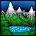Contributor I

I assume you mean C22 for your last coefficient.

On one of my systems I got

A0=2156.625000
B1=-2.528809
B2=-1.034241
C12=0.000876
C11=0.000000
C22=0.000000

result 101.802 kPa.  So that comes out at 1018.02 mBar.

Looking at the  values it seems that the one which is likely to be wrong is C12. Your number is much smaller than what I get.  If you put my numbers into your calculation function do you get the same result as I did?

Also check the decimial point position in B2 your number is a factor of 10 smaller than mine.

Don't forget too that you have to scale the pComp value.  The output is 0-1023 which is for a scale of 50kPa to 115kPa. So range is 65kPa in 1023 steps. Final pressure = (65/1023*pComp)+50

570 Views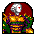Contributor I

Peter, please could you explane how you have calculated C12. The AN3785 says dec pt zero pad=9. So 9 "0" after "."  If I do so I can't get an acceptable result. Even all other values are almost the same as yours.

Thank you!

Michael

570 Views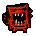Contributor I

Hello,

I have a problem with the MPL115A2.

The coef data are:

3a 4d ba cc bf a6 31 78 00 00 00 00

I calculate:

a0 = 1865.63

b1 = -2.16

b2 = -1.01

c12 = 0.00075

c11 = 0

c22 = 0

Pcomp = -411 (negative!!!)

Any idea?

Many thank !

Nicola (Italy)

570 Views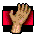Contributor I

Hi Mommmo,

Did you solve your problem?, As they give me similar results.

Negative pressure and a value of PADC = 990

regards

570 ViewsContributor I

I've the same problem as other users of the MPL115A2 programming...

I can read the data from the registers, however, to get the real pressure and temperature is a long way to go from there,

ok, here's my problems and wishing someone can help me, the following are the data I read from the registers \$00 to \$0f

5a c0 75 c0 39 e3 b9 7a ca 7b 32 cc 00 00 00 00

Please, anyone can help me to decipher the mystery of the above data - I know the first four bytes are the Padc and Tadc value, then the a0-msb, a0-lsb, b1.....c22 etc, however, I don't know how to convert these No. into floating point notation - actually I can't find any 'Freescale' document mention about it.

570 ViewsContributor I

It has been a long time since I worked with this component but I can tell you it works fine once you get the math correct. I found that the equations given in the manual are in correct. Unfortunately I don't remember exactly what the issue was so I went back thru my code looking for differences. The following is the only difference I can find:

The manual says:

siPcomp = ((S16)lt3>>13);

Change this to this:

siPcomp = ((S16)(lt3>>13));

Hope this helps

570 Views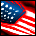Contributor I

lrf wrote:

The manual says:

siPcomp = ((S16)lt3>>13);

Change this to this:

siPcomp = ((S16)(lt3>>13));

That was a HUGE help to me.  Thank you very much!

(This, plus the undocumented ox01 I2C   write that is necessary to initiate the pressure/Temp conversion)

570 ViewsContributor I

Hi

has anyone solved this math mystery? I can finaly read the data from the sensor, but i can't pass thru the math described in the app. note on pages 17-18. As siPcomp i get about 5726, which is significantly more than 1023...

The coefs are fine (proccessed to float just for info:

A0 Result = 2101

B1 Result = -2.4163818359375

B2 Result = -1.2173461914062

C12 Result = 0.00083708763122559

C11 Result = 0

C22 Result = 0

The code is exactly copy-pasted from the app. note, but the result is completly insane...

Has anyone a working code snippet?

My data are here (pressure, temperature, coeffs):  5B 40 81 80 41 A8 B2 AD B2 17 36 DC 00 00 00 00

My code is here:

`unsigned char mpl115a_raw_data;unsigned int mpl115a_p;unsigned int mpl115a_t;signed int mpl115a_a0;signed int mpl115a_b1;signed int mpl115a_b2;signed int mpl115a_c12;signed int mpl115a_c11;signed int mpl115a_c22;//====================================================// MPL115A Placing Coefficients into 16 bit variables//====================================================void mpl115a_raw_dissection(void){ mpl115a_p = (unsigned int)mpl115a_raw_data <<8; mpl115a_p += (unsigned int)mpl115a_raw_data & 0x00FF;  mpl115a_t = (unsigned int)mpl115a_raw_data <<8; mpl115a_t += (unsigned int)mpl115a_raw_data & 0x00FF;  mpl115a_a0 = (signed int)mpl115a_raw_data <<8; mpl115a_a0 += (signed int)mpl115a_raw_data & 0x00FF;  mpl115a_b1 = (signed int)mpl115a_raw_data <<8; mpl115a_b1 += (signed int)mpl115a_raw_data & 0x00FF;  mpl115a_b2 = (signed int)mpl115a_raw_data <<8; mpl115a_b2 += (signed int)mpl115a_raw_data & 0x00FF;  mpl115a_c12 = (signed int)mpl115a_raw_data <<8; mpl115a_c12 += (signed int)mpl115a_raw_data & 0x00FF;  mpl115a_c11 = (signed int)mpl115a_raw_data <<8; mpl115a_c11 += (signed int)mpl115a_raw_data & 0x00FF;  mpl115a_c22 = (signed int)mpl115a_raw_data <<8; mpl115a_c22 += (signed int)mpl115a_raw_data & 0x00FF;}//===================================================//Coefficient 9 equation compensation//===================================================unsigned long mpl115a_compensated_pressure(void){ //Variable used to do large calculation as 3 temp variables in the process below signed long lt1, lt2, lt3, si_c11x1, si_a11, si_c12x2, si_a1, si_c22x2, si_a2, si_a1x1, si_y1, si_a2x2;;  //Variables used for Pressure and Temperature Raw. unsigned int uiPadc, uiTadc;  signed int siPcomp; unsigned long decPcomp;   uiPadc = mpl115a_p >> 6; uiTadc = mpl115a_t >> 6; //******* STEP 1 c11x1= c11 * Padc lt1 = (signed long) mpl115a_c11; lt2 = (signed long) uiPadc; lt3 = lt1 * lt2; si_c11x1 = (signed long) lt3;  //******* STEP 2 a11= b1 + c11x1 lt1 = ((signed long) mpl115a_b1) << 14; lt2 = (signed long) si_c11x1; lt3 = lt1 + lt2; si_a11 = (signed long) (lt3 >> 14);   //******* STEP 3 c12x2= c12 * Tadc lt1 = (signed long) mpl115a_c12; lt2 = (signed long) uiTadc; lt3 = lt1 * lt2; si_c12x2 = (signed long) lt3; //******* STEP 4 a1= a11 + c12x2 lt1 = ((signed long) si_a11) << 11; lt2 = (signed long) si_c12x2; lt3 = lt1 + lt2; si_a1 =(signed long) (lt3 >> 11); //******* STEP 5 c22x2= c22 * Tadc lt1 = (signed long) mpl115a_c22; lt2 = (signed long) uiTadc; lt3 = lt1 * lt2; si_c22x2 = (signed long) lt3; //******* STEP 6 a2= b2 + c22x2 lt1 = ((signed long) mpl115a_b2) << 15; lt2 = ((signed long) si_c22x2) >> 1; lt3 = lt1 + lt2; si_a2 = ((signed long)lt3) >> 16; //******* STEP 7 a1x1= a1 * Padc lt1 = (signed long) si_a1; lt2 = (signed long) uiPadc; lt3 = lt1 * lt2; si_a1x1 = (signed long) lt3; //******* STEP 8 y1= a0 + a1x1 lt1 = ((signed long) mpl115a_a0) << 10; lt2 = (signed long) si_a1x1; lt3 = lt1 + lt2; si_y1 = ((signed long)lt3) >> 10; //******* STEP 9 a2x2= a2 *Tadc lt1 = (signed long) si_a2; lt2 = (signed long) uiTadc; lt3 = lt1 * lt2; si_a2x2 = (signed long) lt3; //******* STEP 10 pComp = y1 +a2x2 lt1 = ((signed long) si_y1) << 10; lt2 = (signed long) si_a2x2; lt3 = lt1 + lt2; // FIXED POINT RESULT WITH ROUNDING: siPcomp = (signed int)(lt3 >> 13); // goes to no fractional parts since this is an ADC count.  //decPcomp is defined as a floating point number. //Conversion to Decimal value from 1023 ADC count value. ADC counts are 0 to 1023. Pressure is 50 to 115kPa correspondingly. //decPcomp = (((unsigned long)siPcomp) * 65000 + 51150000) / 1023; // result in Pa, not kPa  decPcomp = (unsigned long)siPcomp; // temporary return decPcomp;}`

570 ViewsContributor I

Ok, finally i found the problem. The problem is in data type int. I meant that it has 16 bits (as in gcc, borland c, ...) but in fact it has 32. And therefore the negative numbers aren't negative...

The solution is to change all int to short (at least in CW 6.3).570 ViewsContributor I

irf,

'It has been a long time since I worked with this component but I can tell you it works fine once you get the math correct...'

The problem is I can't get the math correct, I need your help, would you please explain how to convert the coef' value into floating point number in plain english, not c programming notation.

eg, the numbers I read from the registers of \$00 - \$0f are:

5a c0 75 c0 39 e3 b9 7a ca 7b 32 cc 00 00 00 00

cw.

570 ViewsContributor I

CW,

Why do you need to understand the math? If you are trying to get the chip to do something then just use the programming that Freescale provided in AN3785, with the small mod I stated above. To understand the math simply reverse engineer the equations.

The chip is what it is and the way they chose to do the calculations is what they chose. Doing the equations at the MC is much faster and open to user tweaking better then having the chip do the math and you are stuck with the results.  Either use it or find another.

My weather station has been running for about a year and without any issues. Its readings track with the national weather service spot on for barometric pressure, given a correction for altitutde (NWS values are at sea level).

570 Views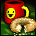Contributor I

Yes I meant C22. I calculated the pressure using your values and got the same result as you. And I think I finally realized what I did wrong. I looked at the first post of this thread and converted the coeffs following that as an example. So C12 is S0.0000000001110001110000 -> 2^-10 + 2^-11 + 2^-12 + 2^-16 + 2^-17 + 2^-18 = 0.0017281. But the sign bit is 1 (0x38E0). Should I invert the 13 fractional bits and add 1 to get the real result? And for the B2 it's probably the same error. Thanks for your help PJH!

570 ViewsContributor I

Can anyone tell me how to convert the uiTADC number to c or F? I'm getting around a 523. What is the conversion factor?

Thansk

Ringo

570 ViewsContributor I

What kind of accuracy/repeatability is anyone getting? Mine is bouncing all over the place.

Alt feet: 149
Alt feet:  57
Alt feet:  97
Alt feet:  57
Alt feet:  97
Alt feet: 109
Alt feet:  57
Alt feet:  45
Alt feet: 109
Alt feet:  45
Alt feet: 109
Alt feet:  57
Alt feet:  57
Alt feet:  97
Alt feet: 109
Alt feet:  97
Alt feet: 109
Alt feet:  57
Alt feet: 109
Alt feet: 109
Alt feet: 109
Alt feet:  97
Alt feet: 109
Alt feet:  97
Alt feet:  45
Alt feet:  57
Alt feet: 109
Alt feet:  97
Alt feet:  57

570 ViewsContributor I

Ringo,

1. The temperature ADC does not have a direct conversion to an actual temperature. You could always try and vary the ambient temperature of the sensor and see what the ADC value is over a range of temperatures. Then you might be able to figure out a conversion factor.

2. The final pressure reading is noisy and thus any conversion of that value to an altitude will also be noisy.  Typically the pressure varies quite slowly so the best approach is to take multiple readings and have a running average of the last measurements so that noise is averaged out.  For our application we take measurements every second and average over the last 100 seconds.

Peter